### Introduction to Neural Networks

When it comes to vision, a computer and a brain start from different baselines. A computer "can only think in numbers," which is to stress the fact that the computer is starting with none of the evolutionary tools that the brain relies on.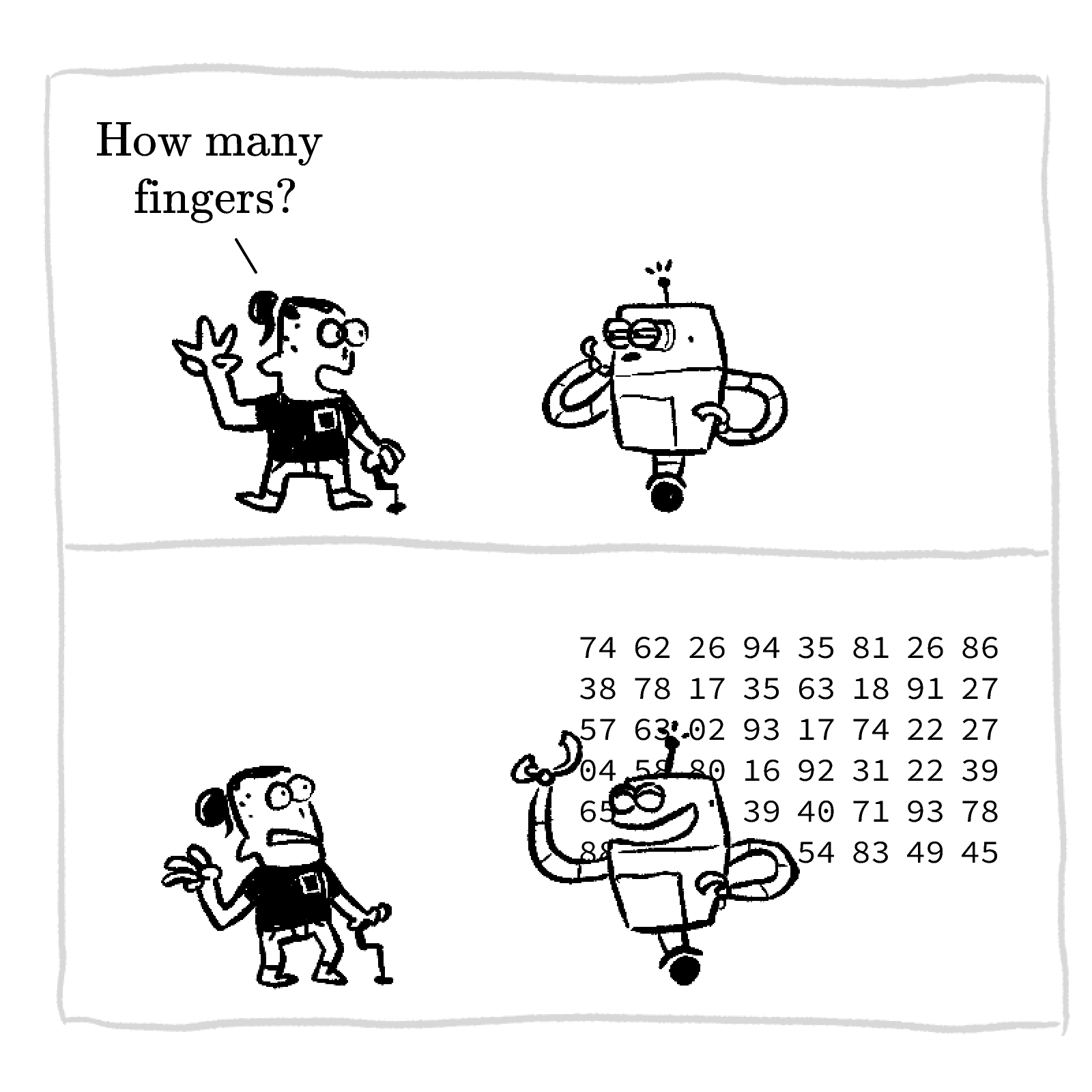Whatever inferences an AI makes about objects, their identity, their relationships, or their movements ultimately has to derive from the information in pixels.

In this quiz, we'll try to design a program that performs an image recognition task. By the end, we'll abandon an algorithmic solution to the vision problem in favor of a more flexible paradigm: neural networks.

# The Folly of Computer Programming

The Simple Shapes dataset consists of $3000$ images of either a triangle, a square, or a circle. Our goal in this quiz is to design a program that can decide which shape is depicted in a randomly selected image from this dataset.

Each image consists of $20\times 20=400$ pixels. At this resolution, the shapes have some irregularities, but a human can still classify them correctly. Here is a sample of images from the dataset.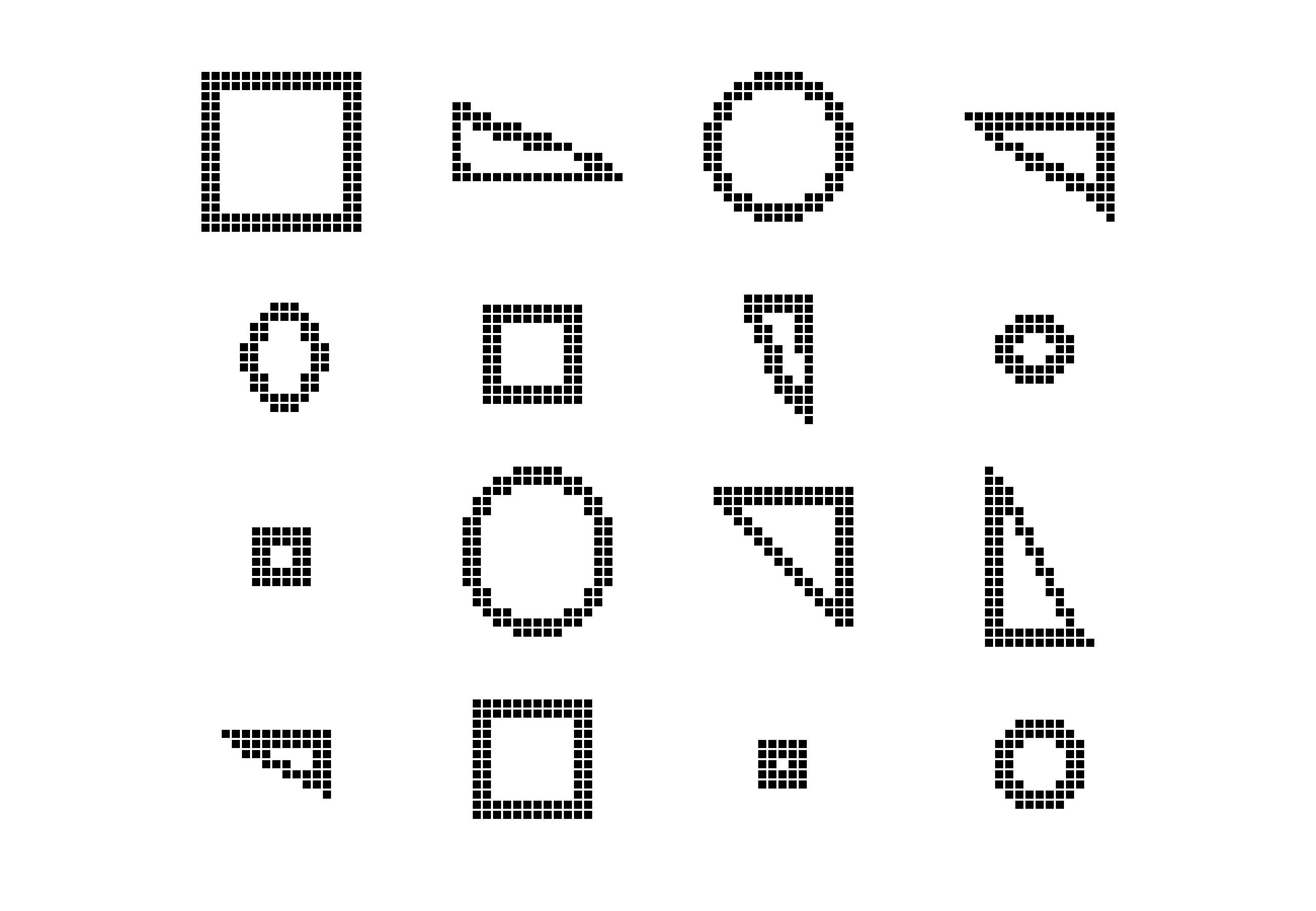What are some dependable differences among the three shapes that we might exploit to design a program that can recognize them reliably?

# The Folly of Computer Programming

If we can program a computer to identify corners in an image, then it can classify shapes by counting the corners. But identifying corners from pixel values alone isn't easy.

In the last exploration, we learned that pixels in a grayscale image are decimals between $0$ (black) and $1$ (white). Here's a pixel array that, at first glance, may contain a corner: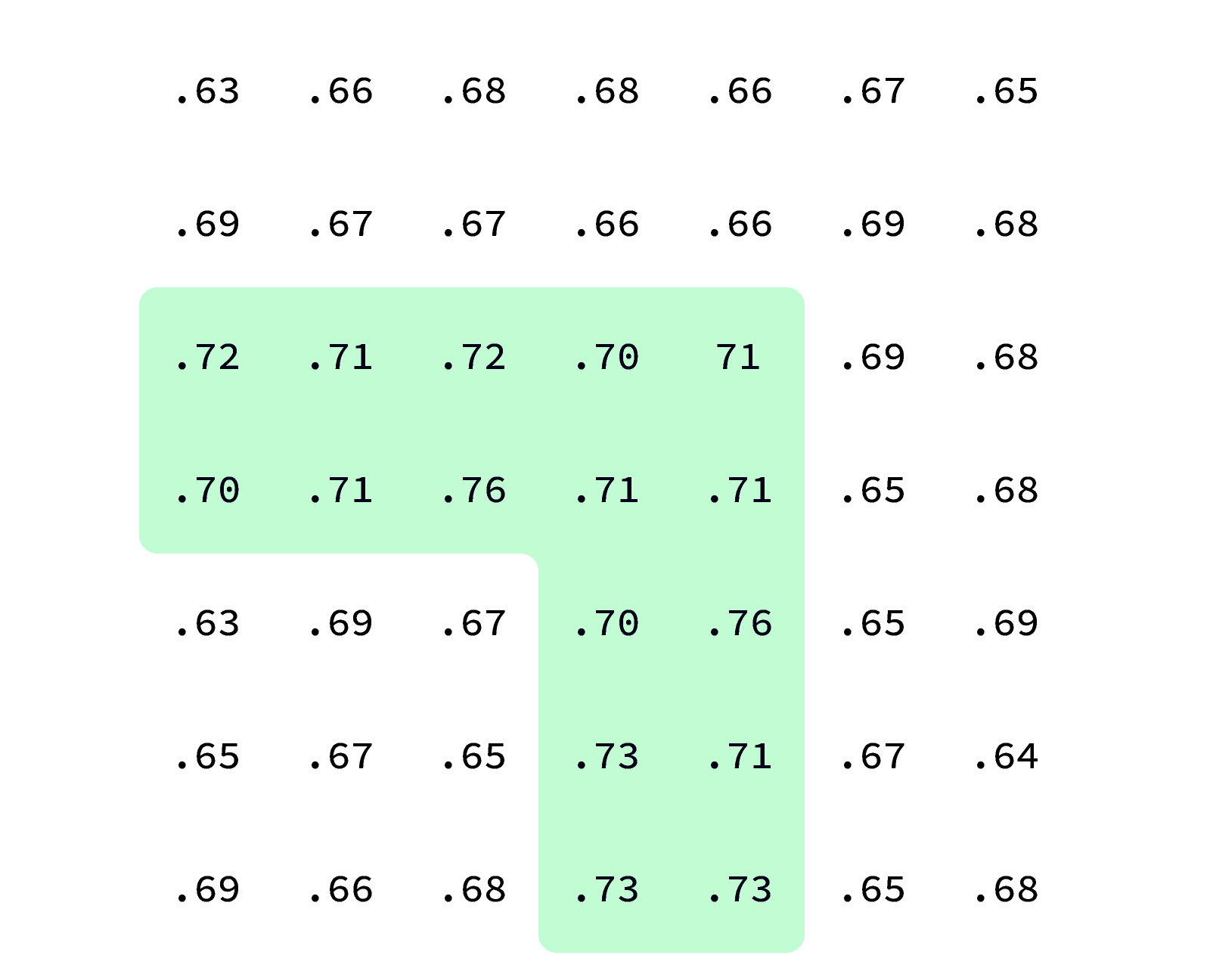However, when we convert this array to a grayscale image, it's hard to see anything at all: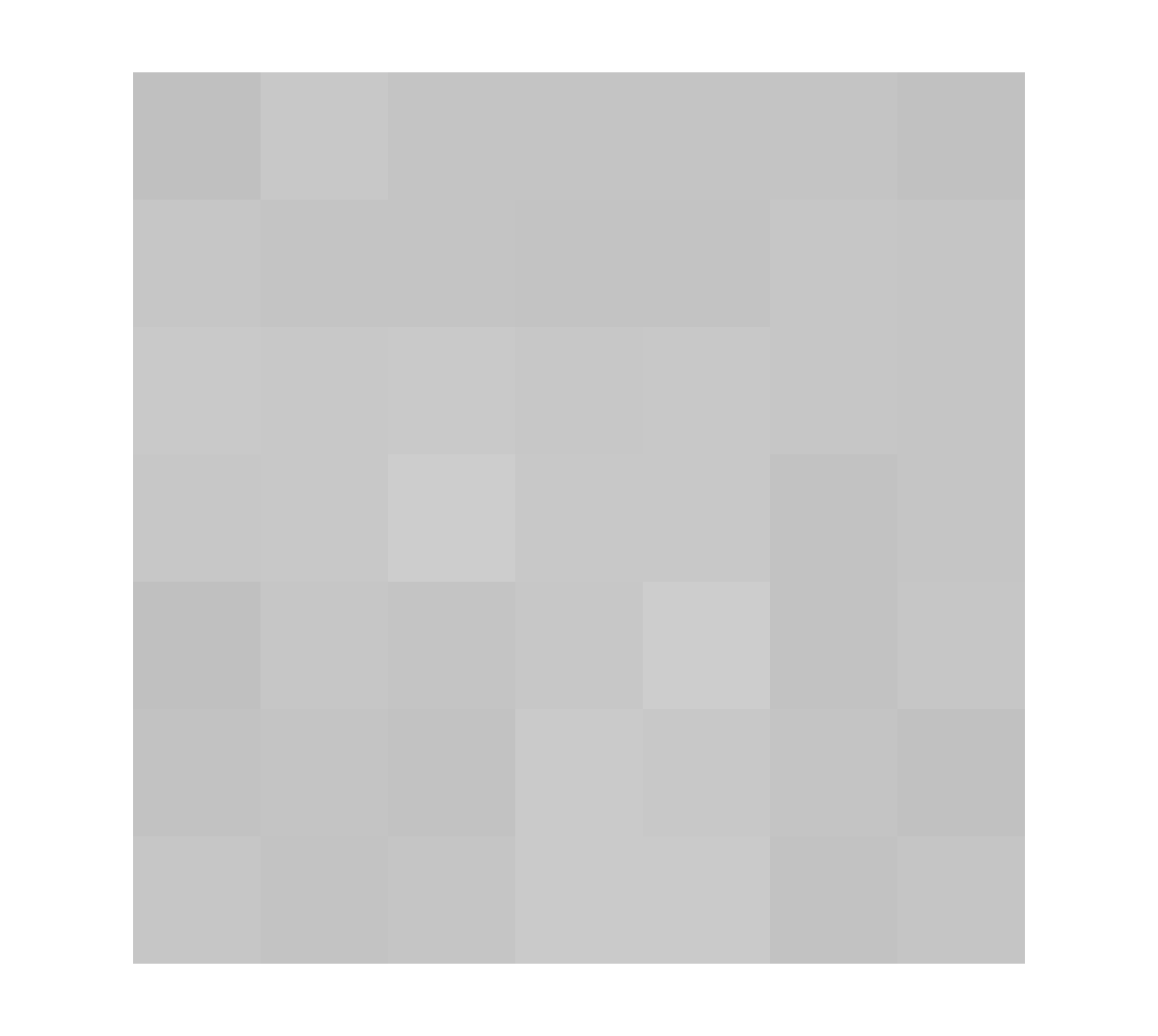To avoid issues like this, all of the shape images are transformed so they have only two possible pixel values: black or white. This is called a binary image. All pixels below some cutoff pixel value are set to black, and the rest are made white.

# The Folly of Computer Programming

One of the simplest ways to find corners is to look at each dark pixel in an image, and count the number of neighboring pixels that are the same color.

We've taken the dark pixel at the corner of this square and highlighted it green. How many neighbors of this corner pixel are dark?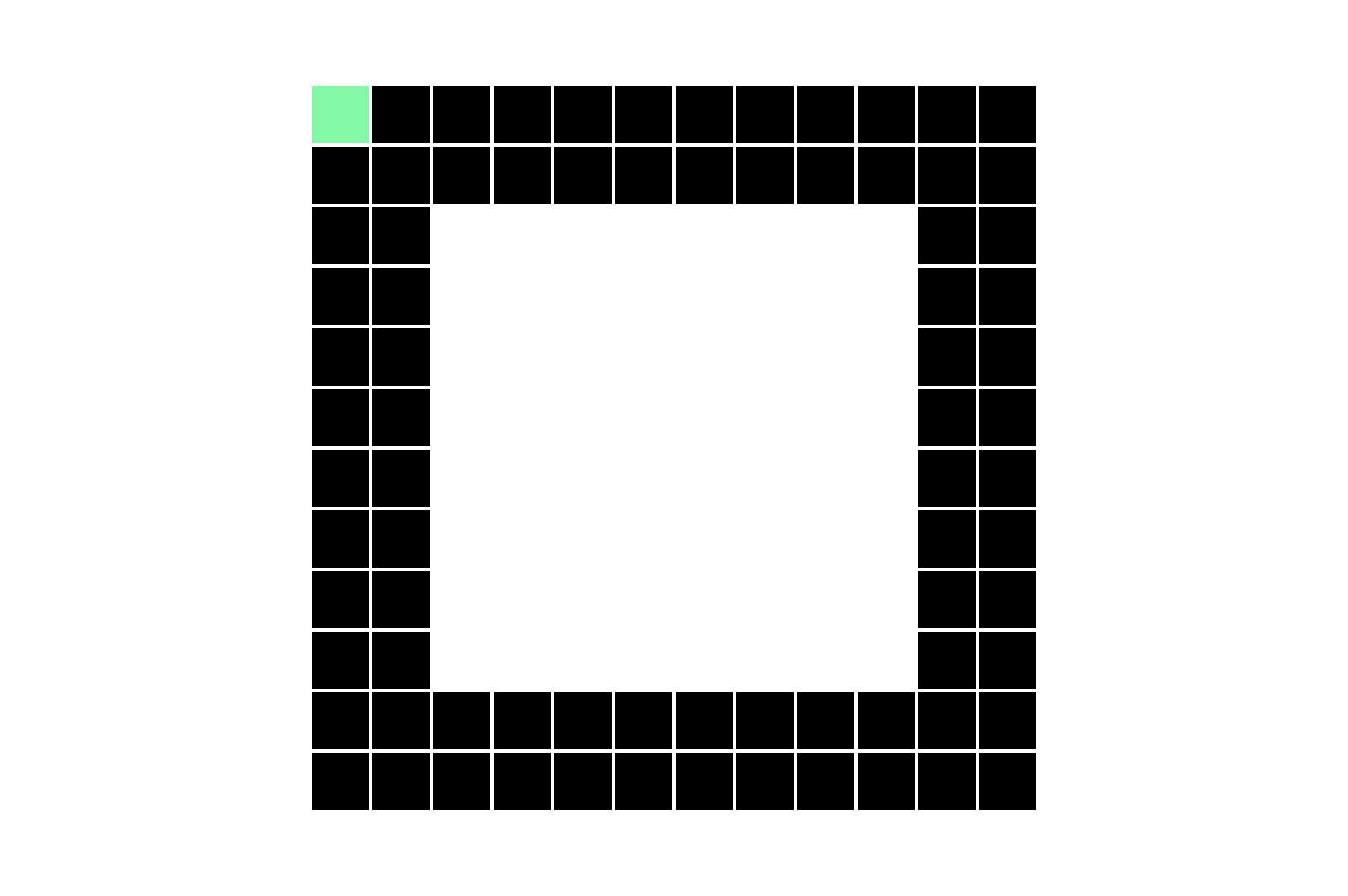Note: Horizontal, vertical and diagonal neighbors count as neighbors.

# The Folly of Computer Programming

If we take a look at a bunch of examples, we can safely say that dark pixels with more than $3$ neighbors are not corners.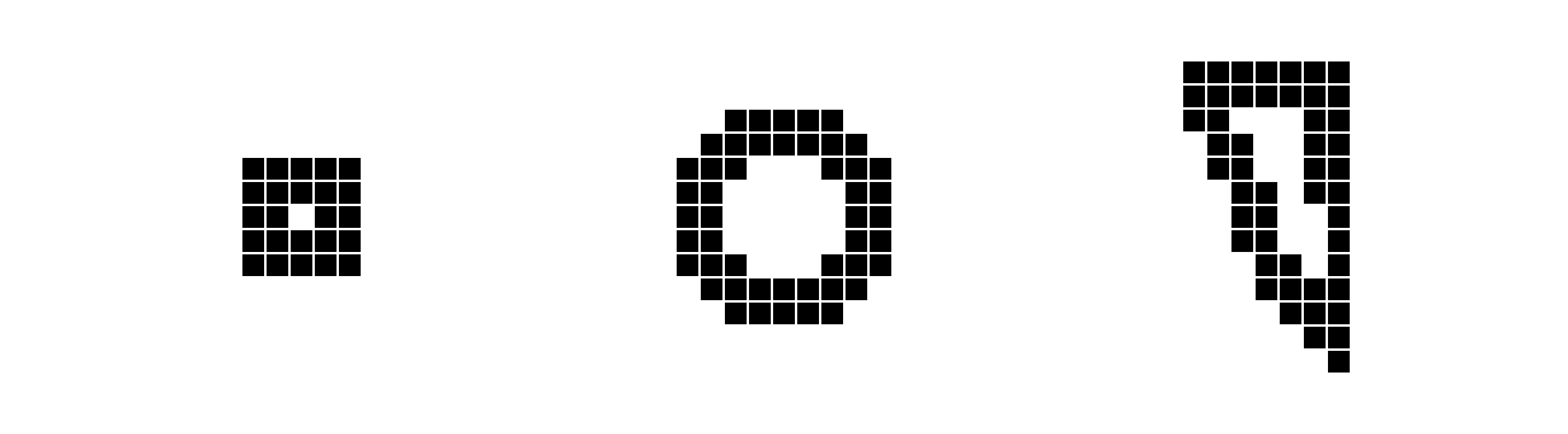Suppose we program a computer to execute the following steps for each shape image we want to classify:

• Visit each dark pixel.
• Count the number of neighboring pixels that are dark.
• If this number is less than or equal to $3,$ then the pixel is a corner.

If the computer follows these steps for every dark pixel, it may detect all of the corners in an image.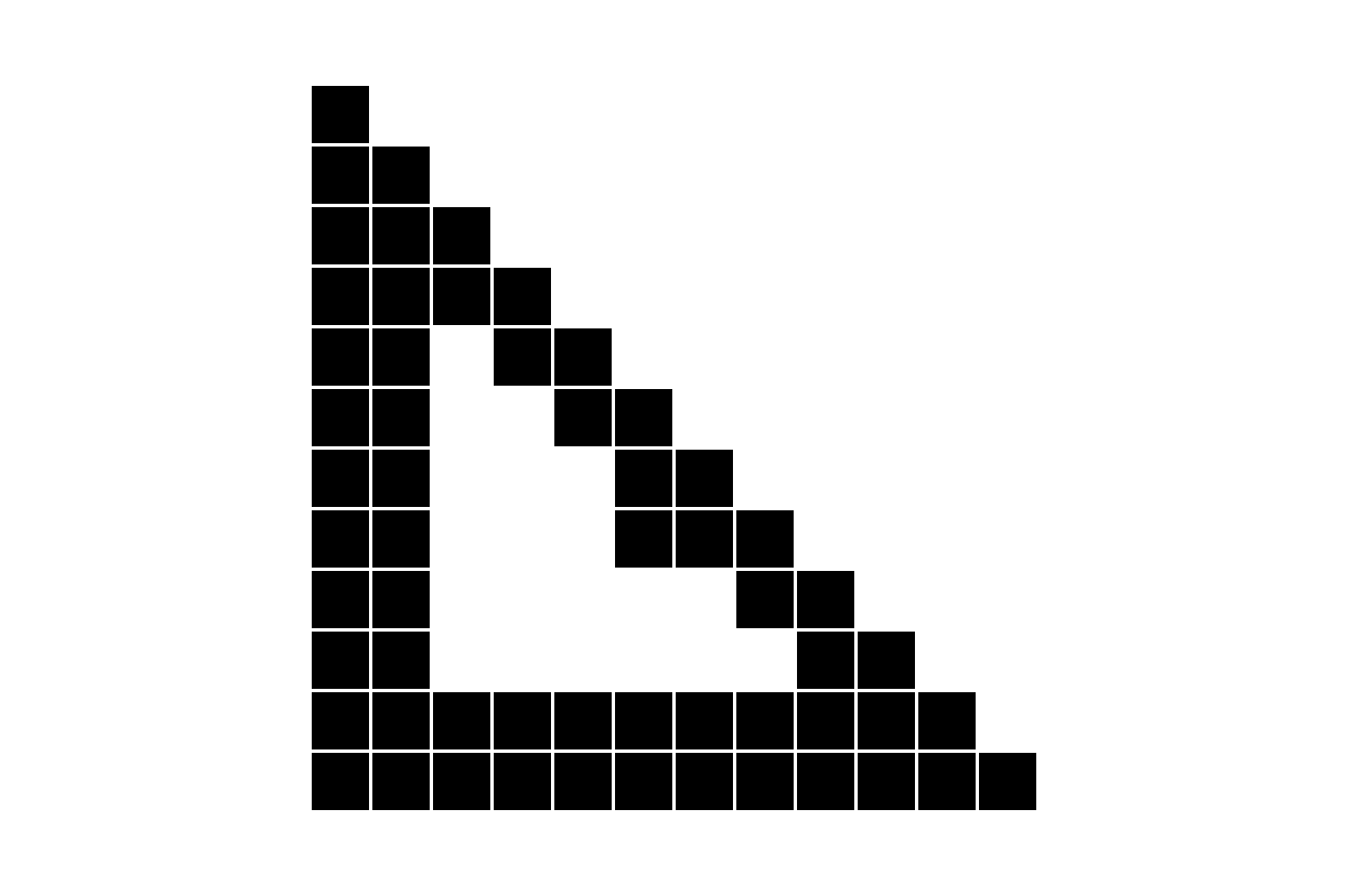Apply these instructions to the image of triangle above. How many corners would it detect?

Remember: Diagonal neighbors count as neighbors.

# The Folly of Computer Programming

The problem with looking at neighboring pixels to decide whether a certain pixel is a corner or not is, if one pixel is out of place, the program may miscount.

We may have better luck with an algorithm that searches for global features rather than making local measurements.

Imagine you are walking around the edge of a pixelated triangle. You are using a compass and tracking your heading (e.g. N, NW, SE) as you walk.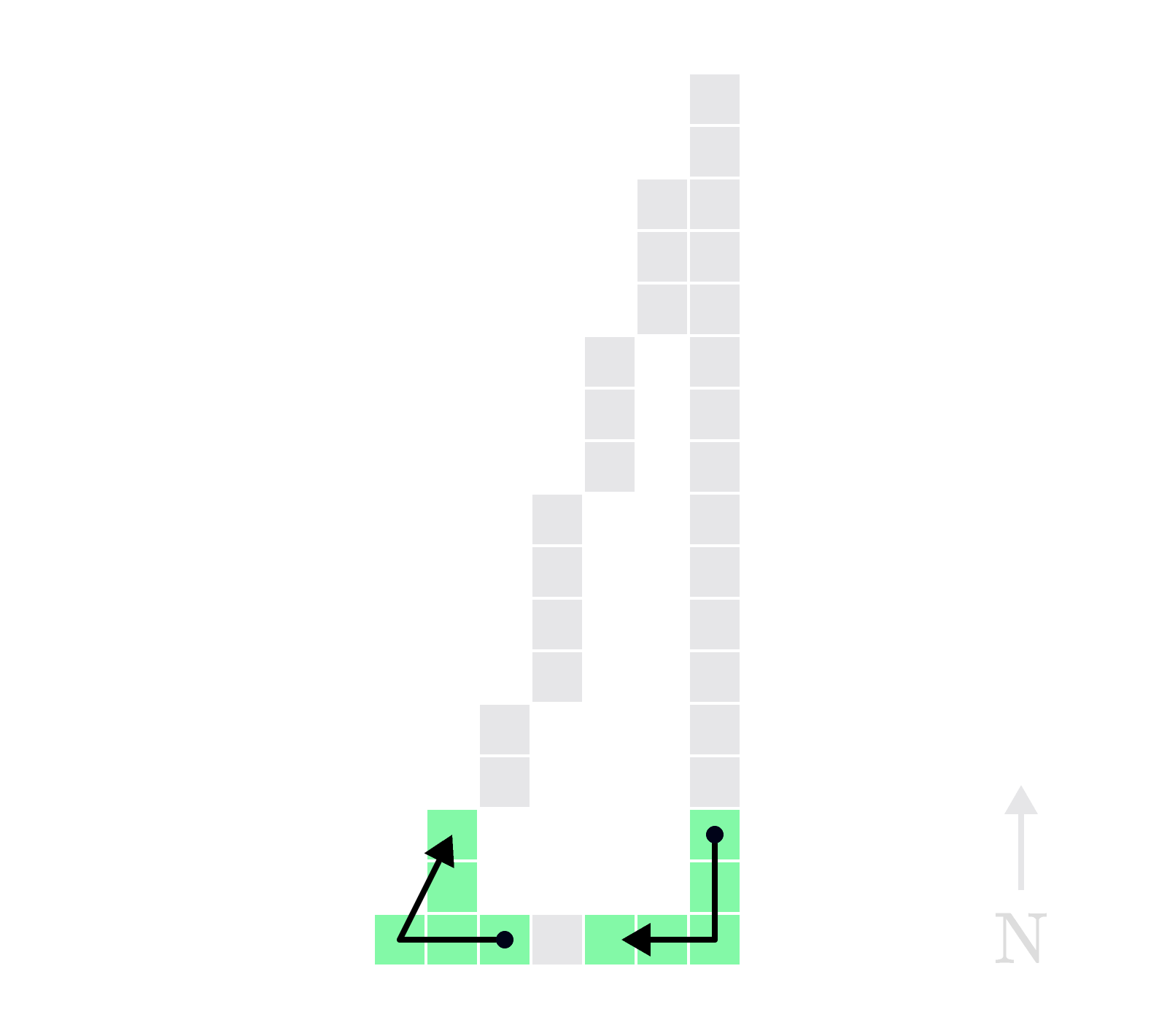At the right angle of the triangle, you are heading South and turn West. This change in heading is $90^\circ.$ Is the change in heading at the next angle you come to smaller or larger than $90^\circ?$

# The Folly of Computer Programming

If we instruct the computer to lay out arrows corresponding to heading as you walk around the edge of the shape, then changes in heading greater than or equal to $90^\circ$ can be counted as corners.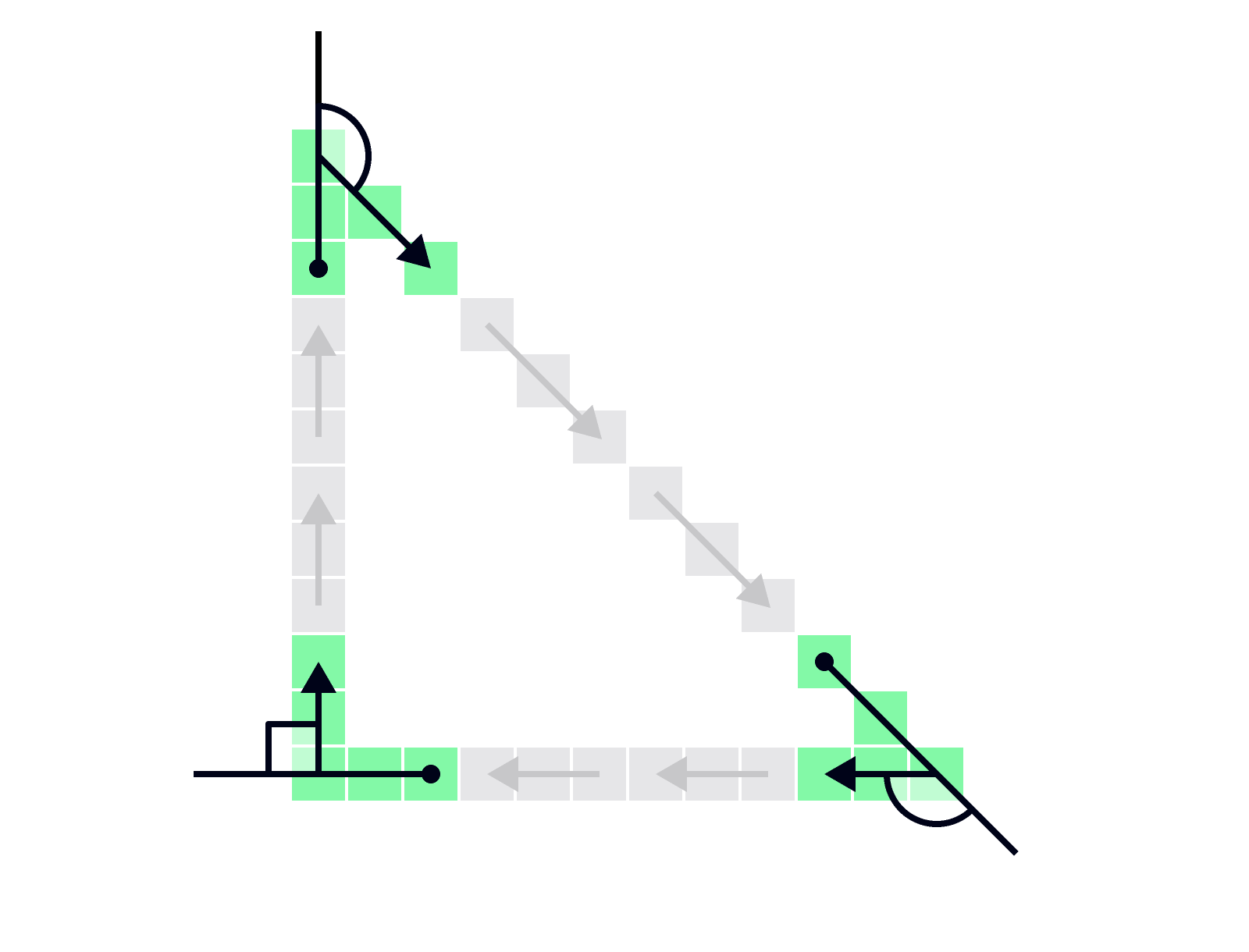Here's a row of sequential heading arrows during edge traversal of a particular shape. Can you tell what kind of shape it is?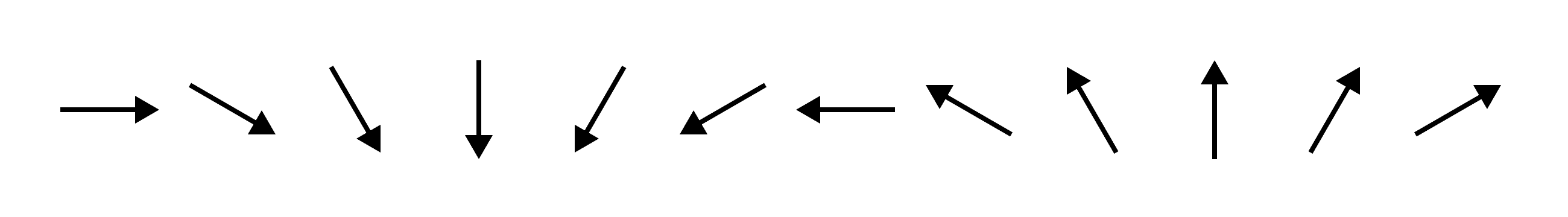# The Folly of Computer Programming

Searching for large changes in heading along the edge of a shape depends on being able to find the heading arrows for any shape. How would a computer do this?

Suppose we search each row and column of the image, starting from the edge and heading toward the center.

The first dark pixel encountered from each direction is marked. This is the edge of the shape.

# The Folly of Computer Programming

The heading arrows are generated by picking an initial edge-pixel and a direction, and then hopping along adjacent edge-pixels until you return to your starting place. On each step, a heading arrow points from the center of edge-pixel you're leaving to the center of the edge-pixel you're hopping to.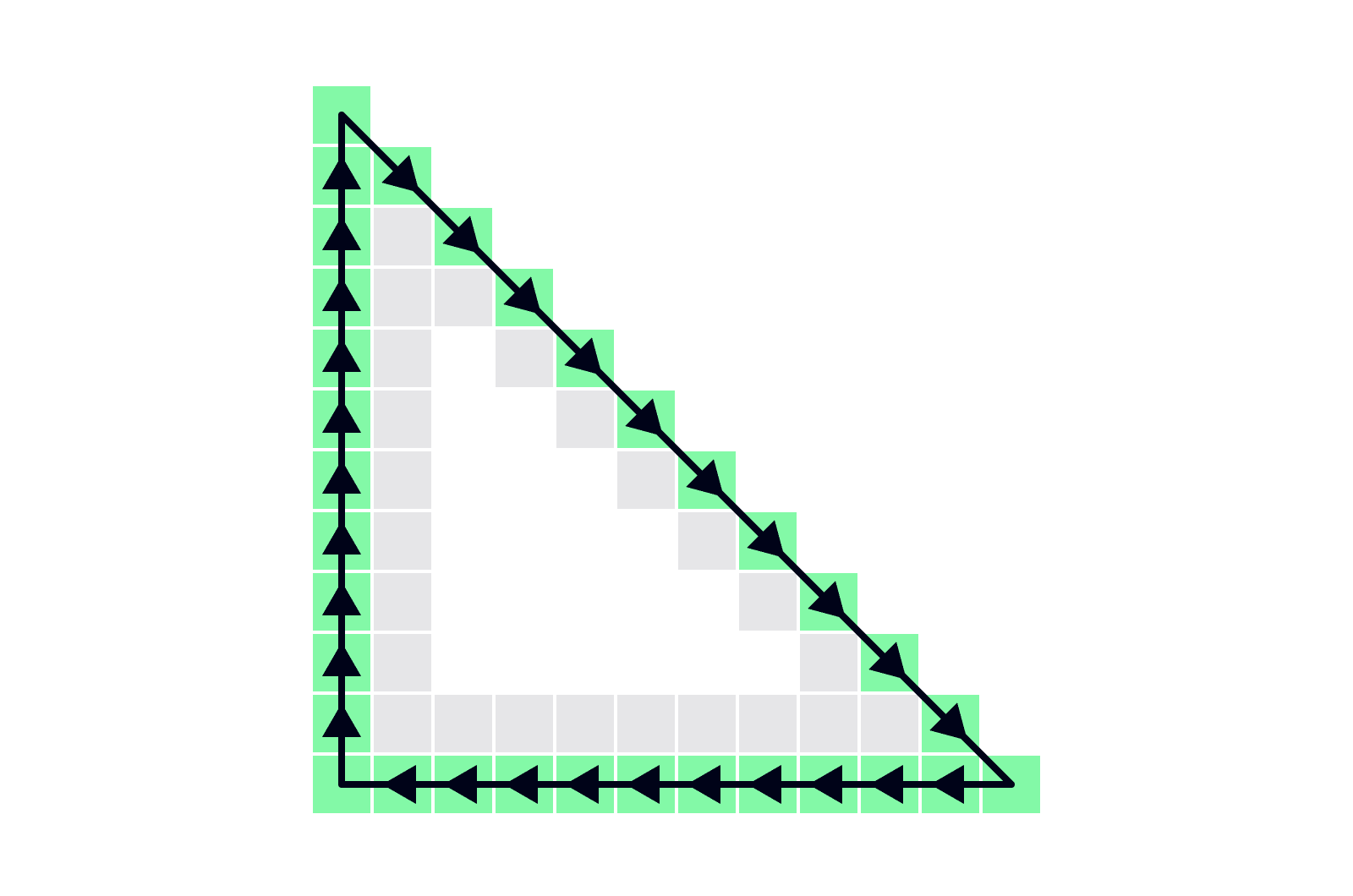When two consecutive heading arrows change by $90^\circ$ or more, the algorithm has detected a corner.

# The Folly of Computer Programming

The triangle below was miscategorized as a square by the neighbor-counting algorithm we tried earlier. Let's see if our new approach can get it right.

If you follow the edge-pixel-stepping algorithm, how many corners would you count as you step along edge-pixels on the shape below?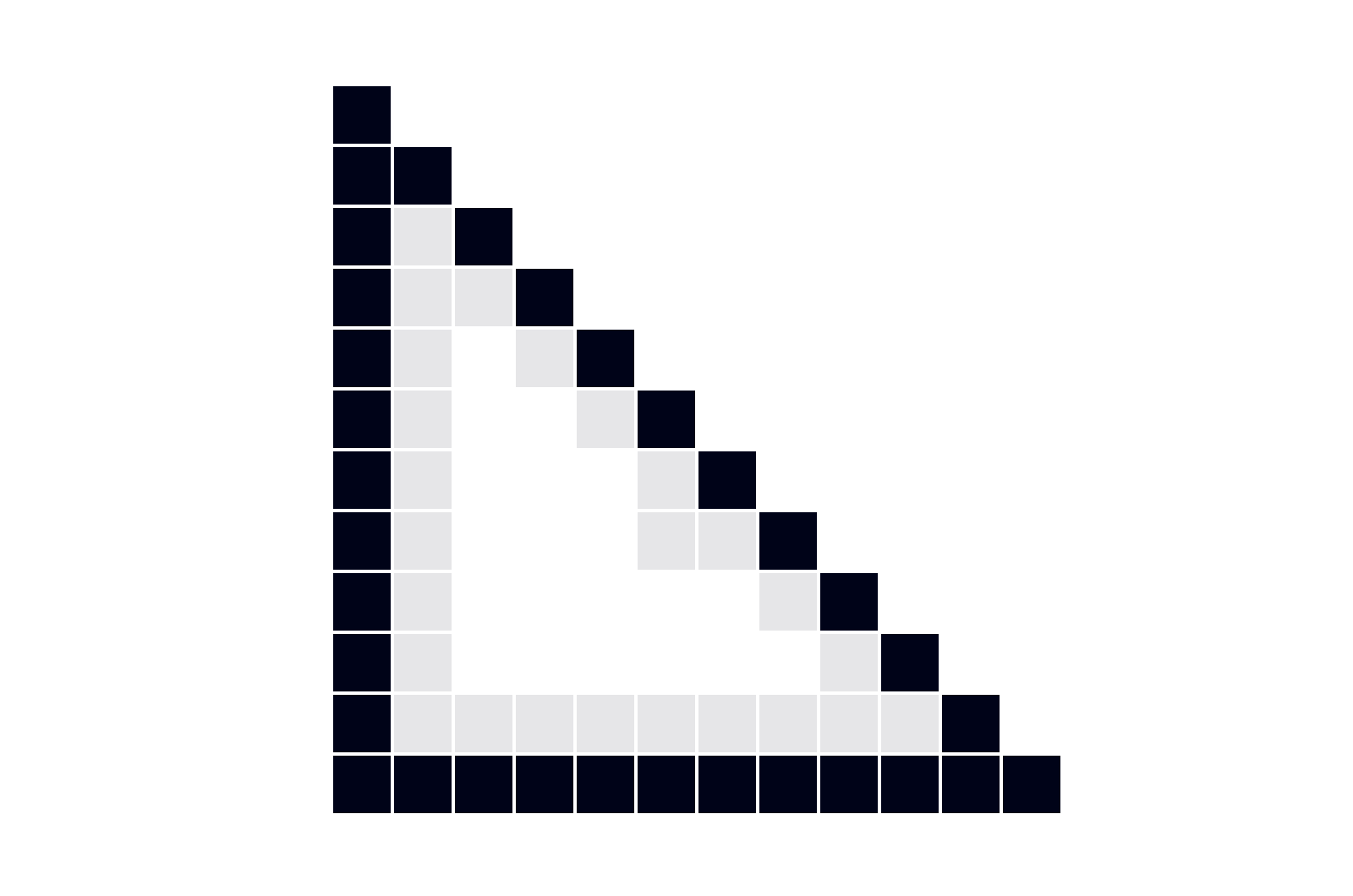# The Folly of Computer Programming

The edge-stepping algorithm passes its first test, but in the Simple Shapes data, examples may have a defect anywhere.

Suppose there's an extra dark pixel attached to the outer edge of the triangle. How many corners are detected on this shape?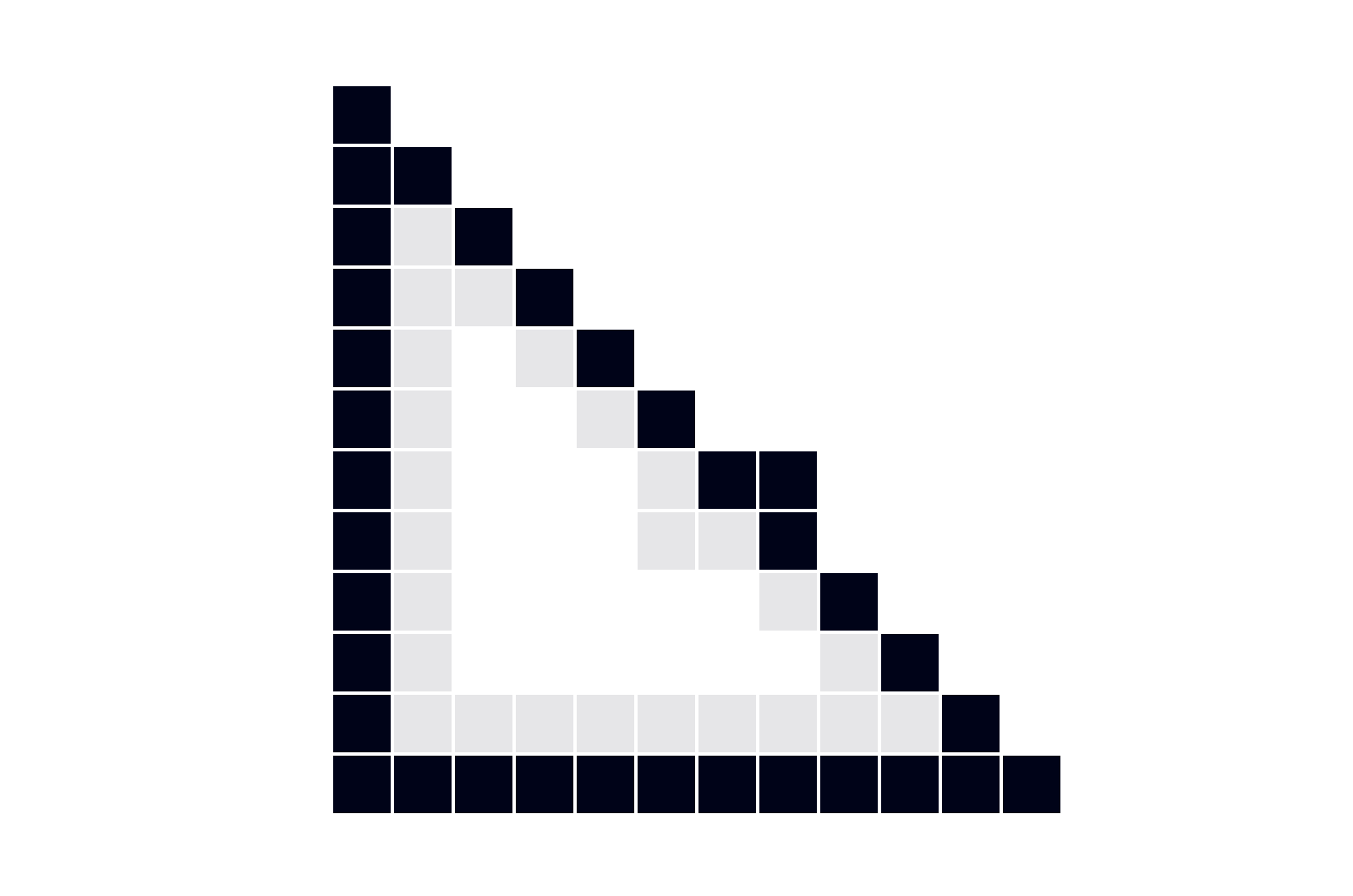# The Folly of Computer Programming

Adding single pixels can throw off the edge-pixel-stepping algorithm, inflating the number of corners detected. One way we might patch over this problem is by omitting some of the rows and columns as we identify the edge-pixels. This would, in some sense, average the headings over larger segments of the edge.

But before we dive in, does the thought of "patching over" this algorithm set off warning bells? It should!

# The Folly of Computer Programming

Suppose you sample the edges using every other row and column, and you find the set of edge-pixels shown as darker pixels on the square below.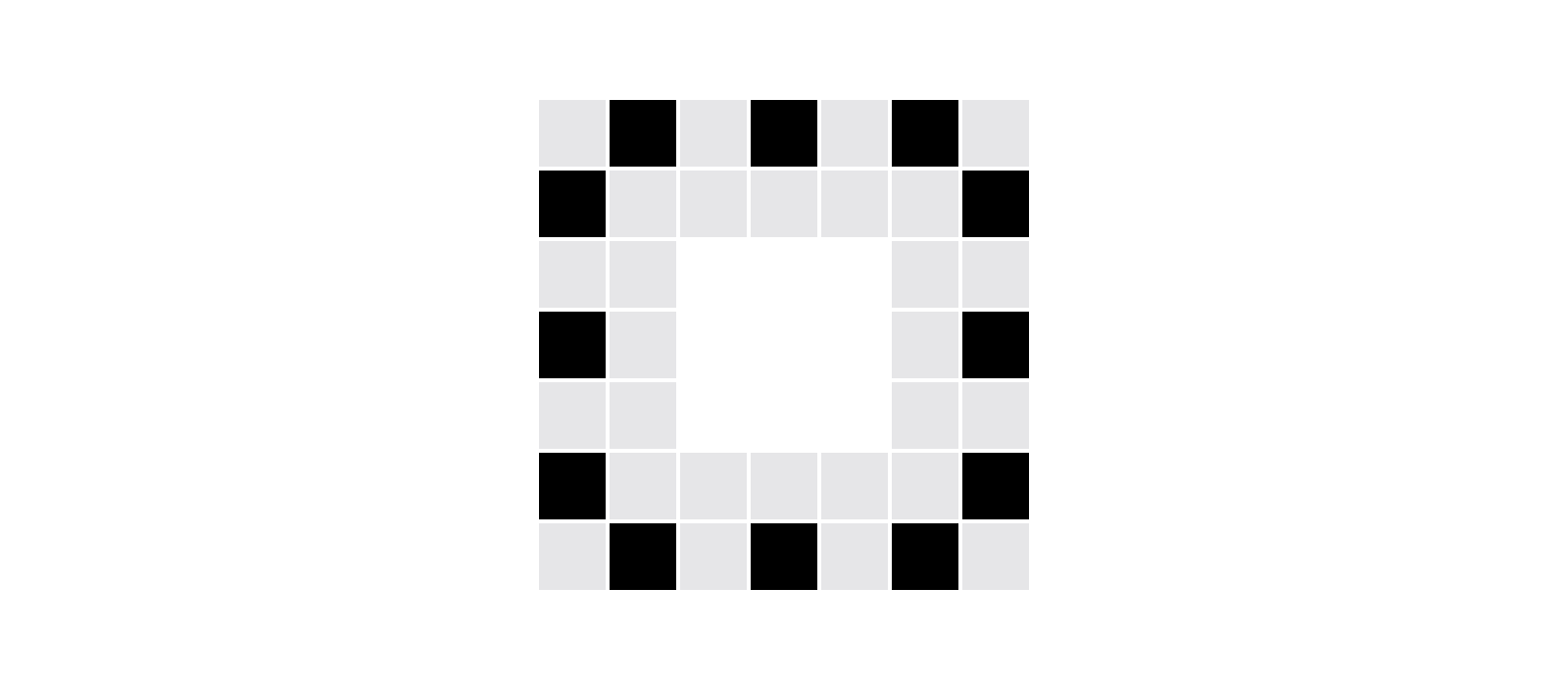How many corners would the edge-stepping algorithm detect?

Remember, a corner is detected when the change in heading between two consecutive heading arrows is $90^\circ$ or greater.

# The Folly of Computer Programming

The edge-traversal algorithm can fail in a number of ways. As we just saw, shapes that are easily identifiable to us are baffling to an algorithm.

You could continue "patching" this algorithm, and you would probably build in enough casework it would eventually perform well on this dataset. But what if you wanted to apply the same program to a new dataset that included stars and parallelograms?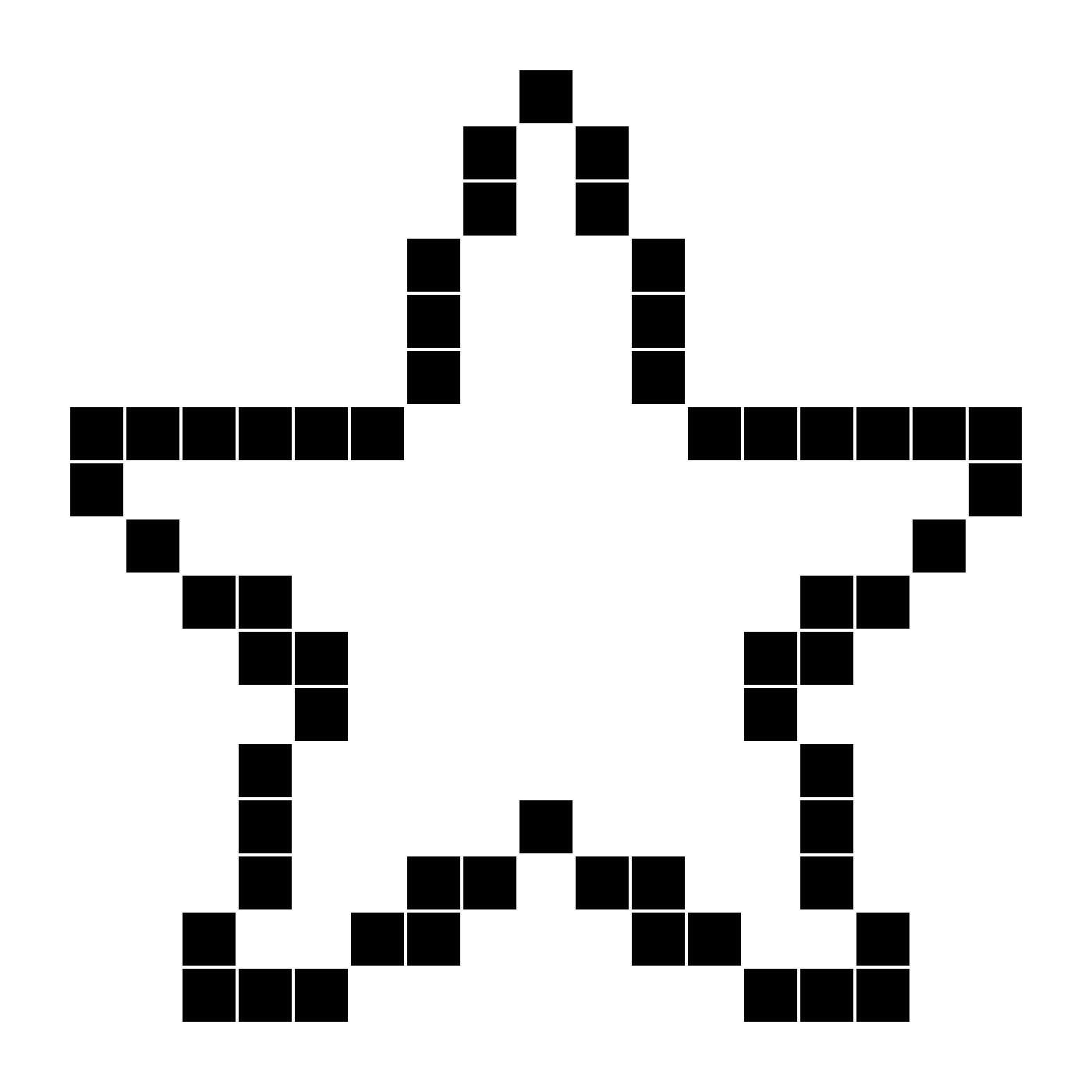Or jungle animals?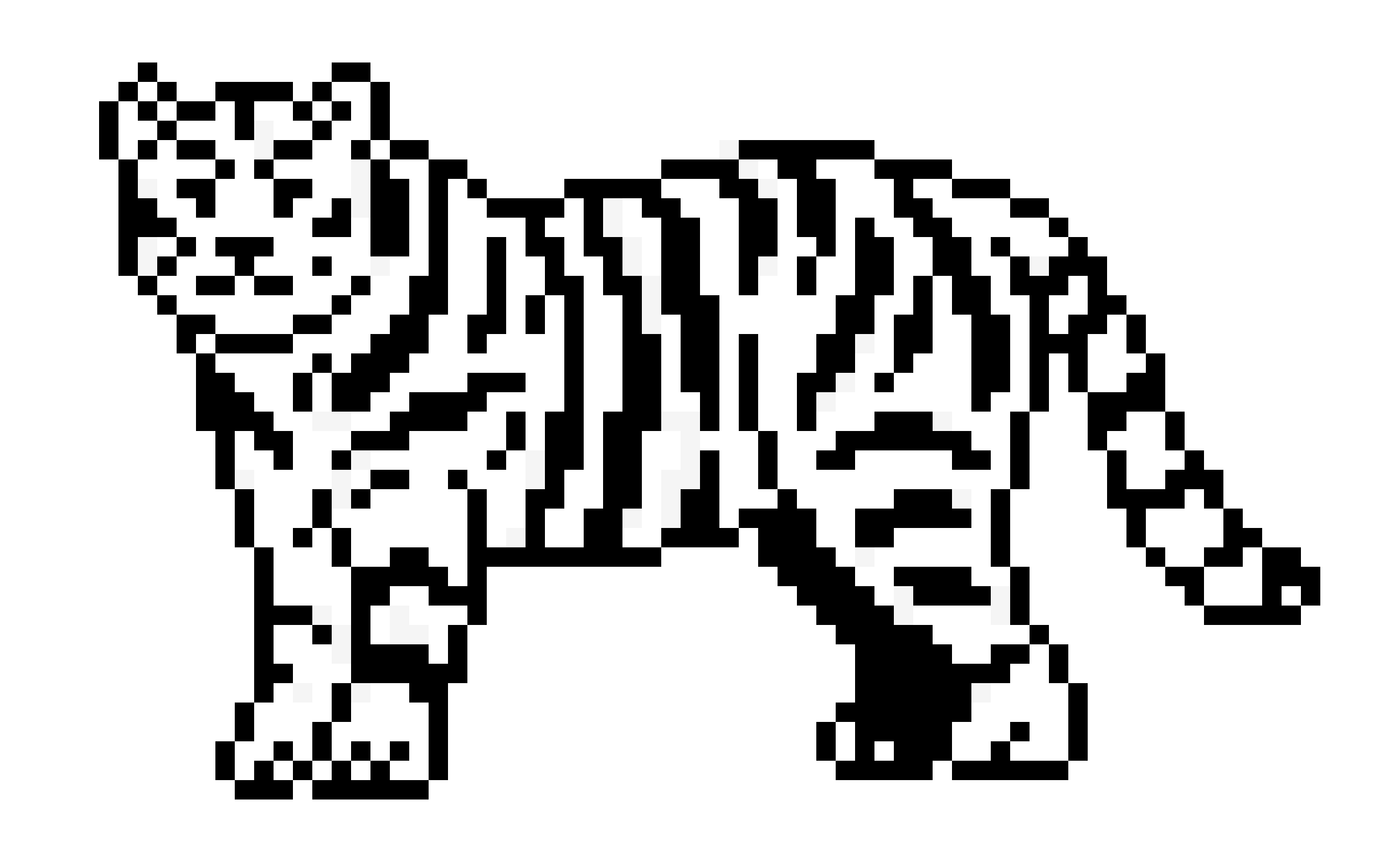Sooner or later, the sane programmer would start looking for a different approach...

# The Folly of Computer Programming

×